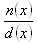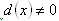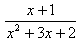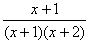Undefined Expressions

A rational expression, where n(x) and d(x) are polynomial expressions is defined when. Since division by 0 is undefined, the expression(s) in the denominator can not equal zero. Values that make an expression in the denominator equal to zero are excluded values.

Example Find excluded value(s) if they exist.Step 1. Factor the expression in the denominator.Step 2. Set each factor in the denominator equal to zero.

x + 1 = 0 or x + 2 = 0

Step 3. Solve each equation.

x = -1 or x = -2

Therefore, -1 and -2 are the excluded values for x.